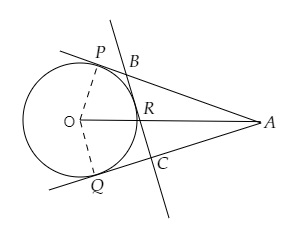# $A$ is a point at a distance $13 \mathrm{~cm}$ from the centre $O$ of a circle of radius $5 \mathrm{~cm}$. $A P$ and $A Q$ are the tangents to the circle at $P$ and $Q$. If a tangent $B C$ is drawn at a point $R$ lying on the minor arc $P Q$ to intersect $A P$ at $B$ and $A Q$ at $C$, find the perimeter of the $\triangle A B C$.

Given:

$A$ is a point at a distance $13 \mathrm{~cm}$ from the centre $O$ of a circle of radius $5 \mathrm{~cm}$. $A P$ and $A Q$ are the tangents to the circle at $P$ and $Q$.

A tangent $B C$ is drawn at a point $R$ lying on the minor arc $P Q$ to intersect $A P$ at $B$ and $A Q$ at $C$.

To do:

We have to find the perimeter of the $\triangle A B C$.

Solution:$\angle OPA = 90^o$    (Tangent at a point on a circle is perpendicular to the radius through the point of contact)

In $\triangle OPA$,

$OA^2 = OP^2 + PA^2$    (By Pythagoras theorem)

$(13)^2 = 5^2 + PA^2$

$PA^2 = 169-25$

$=144$

$=(12)^2$

$\Rightarrow PA = 12\ cm$

Perimeter of $\triangle ABC = AB + BC + CA$

$= (AB + BR) + (RC + CA)$

$= AB + BP + CQ + CA$   (since $BR = BP$ and $RC = CQ$, Tangents from internal point to a circle are equal)

$= AP + AQ$

$= 2AP$        (Tangents from internal point to a circle are equal)

$= 2 \times 12$

$= 24\ cm$

The perimeter of $\triangle ABC$ is $24\ cm$.